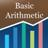# Basic ArithmeticQuantitative Aptitude Questions and Answers section on “Basic Arithmetic” with solution and explanation for competitive examinations such as CAT, MBA, SSC, Bank PO, Bank Clerical and other examinations.

# When a natural number x is divided by 9235, then we get the quotient 888 and the remainder 222, so the value of x is ____?

When a natural number x is divided by 9235, then we get the quotient 888 and the remainder 222, so the value of x is ____? [A]820090 [B]8200920 [C]8200680 [D]8200902 Show Answer 8200902 Because we know that : Dividend = Divisior * Quotient + Remainder Let Dividend is x, then to this above expression => ..

# In 434079, what number should be added so that it can be divisible by 137?

In 434079, what number should be added so that it can be divisible by 137? [A]97 [B]74 [C]75 [D]None of these Show Answer 74 We have to add the difference of remainder and the divisor for make 434079 divisible by 137, (137 – 63 = 74), so option (b) is the right answer.

# From 434079, what number should be subtracted so that it can be divisible by 137?

From 434079, what number should be subtracted so that it can be divisible by 137? [A]173 [B]63 [C]97 [D]Answer can not be determine Show Answer 63 For get the correct answer we have to divide 434079 by 137 and then the obtained remainder 63 which is right answer so option (b) is the right answer.

# How many numbers are there which are divisible by neither 5 nor 7, in the set {200, 201, 202, ….., 798, 799, 800}?

How many numbers are there which are divisible by neither 5 nor 7, in the set {200, 201, 202, ….., 798, 799, 800}? [A]411 [B]412 [C]410 [D]None of these Show Answer 411 Because total numbers in the given set are : (800 – 200) + 1 = 601. Since we have calculated number of numbers ..

# How many numbers are there which are divisible by neither 5 nor 7, in the set {200, 201, 202, ….., 798, 799, 800}?

How many numbers are there which are divisible by neither 5 nor 7, in the set {200, 201, 202, ….., 798, 799, 800}? [A]411 [B]412 [C]410 [D]None of these Show Answer 411 Because total numbers in the given set are : (800 – 200) + 1 = 601. Since we have calculated number of numbers ..